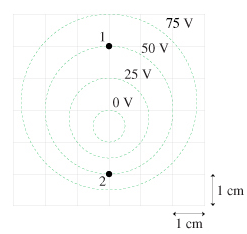# Problem: Part A Determine the magnitude and direction of the electric field at point 1 in the figureE1→=(2500V/m,up) E1→=(7500V/m,up) E1→=(3750V/m,down) E1→=(2500V/m,down)

###### FREE Expert Solution

Electric field:

$\overline{){\mathbf{E}}{\mathbf{=}}{\mathbf{-}}\frac{\mathbf{∆}\mathbf{V}}{\mathbf{d}}}$

ΔV = 50 - 0 = 50 V

d = 2cm (1m/100cm) = 0.02 m

98% (69 ratings)###### Problem DetailsPart A Determine the magnitude and direction of the electric field at point 1 in the figure

E1→=(2500V/m,up)

E1→=(7500V/m,up)

E1→=(3750V/m,down)

E1→=(2500V/m,down)